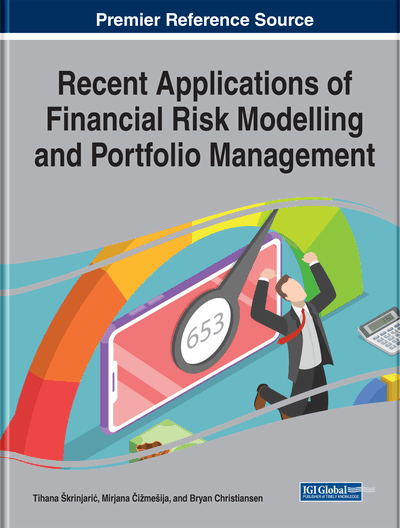# Predicting Equity Returns in Developed Markets

A. Doruk Günaydin (Sabanci University, Turkey)
DOI: 10.4018/978-1-7998-5083-0.ch004
Available
\$37.50
No Current Special Offers

## Abstract

This chapter examines the relation between various firm-specific variables and the cross-section of equity returns in 26 developed countries. Univariate portfolio analyses using equal-weighted returns show that low beta, book-to-market equity, and momentum analysis are also priced in the cross-section of developed market returns, whereas short-term reversal and downside beta manifest themselves in the opposite direction. Univariate portfolio analysis based on value-weighted returns reveal that the predictive power of book-to-market equity and short-term reversal is driven by small stocks. Multivariate firm-level cross-sectional regression analysis document that momentum, short-term reversal, illiquidity, idiosyncratic volatility, hybrid tail risk, lower partial moment are related to expected stock returns. Overall, the most robust cross-sectional predictor in developed market is found to be return momentum.
Chapter Preview
Top

## Introduction

According to the capital asset pricing model (CAPM) of Sharpe (1964), Lintner (1965), and Mossin (1966), market beta should be the only variable that explains the cross-section of equity returns. Although this model has huge impact on the academic research on stock return predictability, current literature has found many firm-specific attributes that add additional explanatory power for expected stock returns. Previous literature has discovered so many variables for explaining the cross-sectional variation in equity returns that Cochrane (2011) called researchers to refine the firm-specific variables that provide unique and statistically significant predictive power for equity return since only systematic factors should provide explanatory power.

This chapter can be regarded as an answer to this call in the developed market environment to identify the cross-sectional return determinants that are correlated with the systematic factors. Literature documents so many variables as potential return determinants in U.S.; however, the evidence outside of the U.S. is incomplete, and this chapter aims to reinvestigate this U.S. evidence in developed markets.

Although there are some studies on the cross-section of equity returns in developed markets, these studies have restrictions in two dimensions. First, they only examine a limited number of potential expected return determinants. Second, they restrict their analysis in a single country or a small group of countries. The main goal of this chapter is to investigate the relation between a comprehensive set of firm-specific attributes that can affect equity returns for a sample of equities from 26 developed countries. Therefore, the reader will be able to examine the unique information that a firm-specific variable provides compared to other variables and compare these results across all developed countries.

This chapter will examine the potential return determinants which can only be extracted from the empirical return distribution of equity returns. These variables are, the market beta, size and value effects (Fama and French (1993)), return momentum (Jegadeesh and Titman (1993)), short term reversal (Jegadeesh (1990)), illiquidity (Amihud (2002)), idiosyncratic volatility (Ang, Hodrick, Xing and Zhang (2006)), co-skewness (Harvey and Siddique (2000)), tail risk proxies such as value-at-risk, expected shortfall, lower partial moment and hybrid tail covariance risk (Bali, Cakici and Whitelaw (2014)), downside beta (Bawa and Lindenberg (1977)), and lottery demand (Bali, Cakici and Whitelaw (2011)). There are various accounting-based variables that are documented to explain the cross-section of equity returns in U.S.; however, it is not quite practical to compare accounting information across these countries due to the sparsity of accounting data and variations in accounting practices in different countries. Therefore, the set of variables are limited to be the ones that can be extracted from the equity return distribution. The only exception is the book-to-market ratio of equity since book value of equity is easier to calculate and compare across counties.

Several methodologies are utilized to test the hypothesis. First, the author forms decile portfolios based on one of the firm-specific attributes and examines whether equities in the highest firm-specific attribute decile generate significant return than those in the lowest firm-specific attribute decile. In addition to univariate portfolio analysis, bivariate and multivariate analysis are implemented to investigate which firm-specific attributes have the power to explain the cross-section of developed market equity return using data from 26 countries.

## Key Terms in this Chapter

Tail Risk: A measure of portfolio or individual asset risk which is three standard deviations higher than the risk of the normal distribution.

Portfolio: A collection of financial assets such as stocks, bonds, currencies, and cash.

Value-Weighted Return: A type of weighting methodology to calculate portfolio return that gives a weight to an asset in the portfolio based on the asset’s market value.

Equal-Weighted Return: A type of weighting methodology to calculate portfolio return that gives the same weight to each asset in the portfolio.

Downside Risk: A measure of risk that the realized return of the portfolio or individual asset is less than the expected return.

Descriptive Statistics: A summary statistics that describes the basic aspects of the data.

Regression: A statistical process for determining the relation between one dependent variable and various other variables (also known as independent variables).

## Complete Chapter List

Search this Book:
Reset